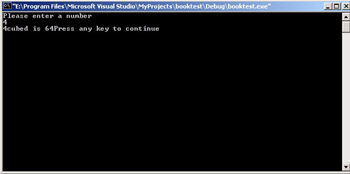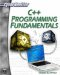So far we have only used header files that were built into C++. We have not used any files that we created. This section will change all of that. Header files are used to contain the prototypes for a function. (Other things can be placed in them, as we will see later in this book.). All you need to do is open your favorite text editor and place the prototype lines in that editor, then save the file with an .h extension.

### Example 4.4

This example will show you how to create and how to use header files for function prototypes.

Step 1: Open your favorite text editor and type in the following code.

`// function prototypes. float cube_number(float num); int cube_number(int num); `

Save this file as test.h.

Step 2: Open a new instance of your favorite text editor and type the following code.

`// Include statements   #include <test.h> #include <iostream>  using namespace std; int main() {  float number;  float number4;  cout << "Please enter a number \n:’  cin >> number;  number4 = cube_number(number);  cout << number << " cubed is " << number4;  return 1;  } int cube_number(int num) {  int answer;  answer = num * num * num;  return answer; } float cube_number(float num) {  float answer;  answer = num * num * num;  return answer; }`

Step 4: When you run your code, you should see something like Figure 4.4.This is a simplified example, but it does illustrate the basic points. Usually the actual functions would also be in a different file, if you were using a header file. However, in this case the purpose was to introduce you to creating your own header files.C++ Programming Fundamentals (Cyberrookies)
ISBN: 1584502371
EAN: 2147483647
Year: 2005
Pages: 197
Authors: Chuck Easttom